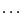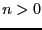Next: How to read this Up: ALIAS-C++ Previous: ALIAS-C++   Contents

Introduction

ALIAS-C++ is a C++ library of algorithms, intended to be run on Sun computers and PC under Unix or Linux, that deal with problems like solving systems of equations and inequalities,optimization, linear algebra. Expressions involved in these problems may be arbitrary combination of the most classical mathematical functions (algebraic terms, sine, cosine, log etc..) and whose coefficients are real numbers or, in some cases, intervals. Most algorithms in ALIAS are based on interval analysis and can be used for almost any system as soon as it is composed of classical mathematical operators (see section 2.1.1.2 for the available operators). Some algorithms may be used only for problems with specific structure such as solving algebraic, linear, distance,equation systems. ALIAS may also deal with functions that involve determinants of matrices, without having to expand the determinants. Without being exhaustive you may find in ALIAS algorithms that enable one to:
• find an approximation of the real roots of 0-dimensional systems
• find an approximation of the variety defined by n-dimensional systems
• find an approximation of the global minimum or maximum of a function (eventually under equations and/or inequalities constraints) up to an accuracy provided by the user
• analyze a system of algebraic equations to determine bounds for its real roots
• parse a formula file describing a set of functions in order to compute an interval evaluation of the functions that can be used for the solving procedures
• check the regularity of an interval matrix
• provide an enclosure of definite integrals
This version of the library has about 86353 lines of C++ code, to which should be added the 13 000 lines for BIAS/Profil/ Most of these algorithms are designed in view of their use in a parallel implementation (see chapter 13).

This library is one of the main software development platform of the COPRIN project. There are various motivations underlying the development of this platform:

• interval analysis allows to solve very different problems (not only solving systems of equations) as soon as the unknowns are restricted to lie within a given domain, which is relevant to many applications. The expertise of the COPRIN project in application domains such as mechanism theory, control theory, robotics has allowed us to develop algorithms and test the library on numerous realistic problems (historically speaking it was the need of tools to solve problems in these fields that has led to the development of ALIAS)
• interval analysis allows to deal with uncertainties that are present in almost all applications
• interval analysis allows to take into account numerical round-off errors that may have a very negative impact on the result of classical numerical analysis algorithms. This allows one to get safe results: whenever an interval-analysis based method will give a solution then the result is guaranteed. On the other hand such algorithm may just states that the current problem cannot be solved with the current computer arithmetics. At the same time interval-analysis based methods may provide an exact answer in the sense that it will be possible to get a solution of the problem wit an arbitrary accuracy in term of number of exact digits for the solution.
• classical problem solving approaches rely on the transformation of the initial problem into a solving problem that have the same solution (or solutions that may be transformed into the solutions of the initial problem) but which has a structure that is more appropriate for solving (for example a system of sine and cosine will be transformed into an algebraic system for which there is powerful solving methods). This operation may transform a simple problem into a much more complex solving problem. At the opposite interval analysis allows one to focus on the problem to be solved which will often results in a more efficient strategy This may also be considered a drawback of interval analysis: very often it is necessary to think backward and to focus on what is really the problem to be solved in view of a solving by interval analysis. We will present some examples of this approach.
• although in many cases other approaches may be used to solve problems addressed in ALIAS they are very often computer intensive. A short pre-processing with interval analysis based methods may avoid using these approaches whenever it is not necessary, therefore drastically reducing the whole computation time. Furthermore the computation time of interval analysis based methods will decrease with the decrease of the search space: hence there will always be a limit on the search space under which the interval analysis method will be faster than any other method
• although they are numerous papers and books on interval analysis (see [5,6,17,18,20,21,24] to name a few) the few implementations that are freely available are far from being complete. Our purpose is to offer a wide range of algorithms
• various communities are working on interval analysis based algorithms: constraint programming, operational research, numerical analysis,Furthermore other communities (such as the algebraic geometry community) are addressing the same problems. In our view there is a lack of collaboration between these communities although basically they intend to solve the same type of problems and that the different methods are very often complementary
• the ALIAS-C++ library is partly interfaced with a Maple package. We strongly believe that symbolic computation, apart for being very convenient to produce error-free C++ code, will also play an important role to improve the efficiency of the algorithms. The ALIAS-Maple manual provides procedures and examples for this increase in efficiency
• we do not claim that interval analysis is an universal tool: it may fail to solve a given problem. It is almost impossible to determine beforehand if interval-analysis based method will work. One of the purposes of ALIAS is to provide the necessary tool to test quickly existing methods. Another purpose is to provide the basic tools so that new algorithms may be developed efficiently. On the other hand interval analysis allow to solve problems for which (to the best of our knowledge) there is no alternate solution.
• we have sometime heard the somewhat negative comment that interval analysis is "only" a version of the branch-and-bound algorithm. Although branch-and-bound is indeed a key component of the interval analysis algorithm we strongly believe that classifying the strategy only by this component is unfair as many other methods are used (and will make a drastic difference in term of computation time). Furthermore we consider that methods should also be judged according to what problem they may solve. In that regard interval analysis is very rich and has proved to be effective in realistic applications problems for which, to the best of our knowledge, there was no alternate methods (chapter 15 will present some of this problems)

ALIAS is partly interfaced with Maple, see the ALIAS-Maple documentation.

This manual contains the following chapters:

• Chapter 2 is devoted to a brief description of interval analysis and to the various algorithms of ALIAS enabling to solve systems, either general purpose solving methods or algorithms for specific type of systems (e.g. system with a large number of linear terms or involving determinant of matrices).
• Chapter 3 deals with the analysis of system of functions
• Chapter 4 deals especially with the analysis of trigonometric functions
• Chapter 5 describes algorithms for the analysis of univariate polynomial.
• Chapter 6 is devoted to the study of parametric polynomials and calculation on the eigenvalues of parametric matrices
• Chapter 7 is devoted to procedure related to linear algebra
• Chapter 8 deals with global optimization problems, constrained or not.
• In Chapter 9 we describe how ALIAS can be used for dealing with one-dimensional systems anddimensional systems
• Chapter 10 is devoted to guaranteed integration procedures
• Chapter 11 describes some miscellaneous ALIAS procedures
• Chapter 12 is devoted to the ALIAS parser and the generic analyzer and solver based on this parser
• In Chapter 13 we explain how ALIAS can be used in a parallel implementation
• In Chapter 14 we explain how to use and install ALIAS,
• the examples treated in the various chapters are presented in chapter 12,
• Chapter 15 presents various examples used in this documentation together with some examples of problems that interval analysis can solve
• Chapter 16 is the troubleshooting chapter, in which is explained what can go wrong with ALIAS procedures and how to adjust ALIAS parameters to obtain a better efficiency

SubsectionsNext: How to read this Up: ALIAS-C++ Previous: ALIAS-C++   Contents
Jean-Pierre Merlet 2012-12-20